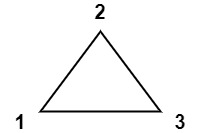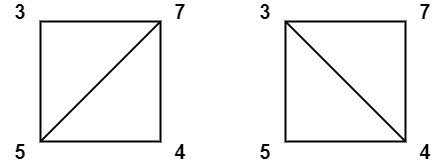# 1039. Minimum Score Triangulation of Polygon

You have a convex `n`-sided polygon where each vertex has an integer value. You are given an integer array `values` where `values[i]` is the value of the `ith` vertex (i.e., clockwise order).

You will triangulate the polygon into `n - 2` triangles. For each triangle, the value of that triangle is the product of the values of its vertices, and the total score of the triangulation is the sum of these values over all `n - 2` triangles in the triangulation.

Return  the smallest possible total score that you can achieve with some triangulation of the polygon.

Example 1:``````Input: values = [1,2,3]
Output: 6
Explanation: The polygon is already triangulated, and the score of the only triangle is 6.
``````

Example 2:``````Input: values = [3,7,4,5]
Output: 144
Explanation: There are two triangulations, with possible scores: 3*7*5 + 4*5*7 = 245, or 3*4*5 + 3*4*7 = 144.
The minimum score is 144.
``````

Example 3:``````Input: values = [1,3,1,4,1,5]
Output: 13
Explanation: The minimum score triangulation has score 1*1*3 + 1*1*4 + 1*1*5 + 1*1*1 = 13.
``````

Constraints:

• `n == values.length`
• `3 <= n <= 50`
• `1 <= values[i] <= 100`

``````class Solution {
public:
int minScoreTriangulation(vector<int>& A) {
int n = A.size();
vector<vector<int>> dp(n, vector<int>(n));
for (int i = n - 1; i >= 0; --i) {
for (int j = i + 1; j < n; ++j) {
for (int k = i + 1; k < j; ++k) {
dp[i][j] = min(dp[i][j] == 0 ? INT_MAX : dp[i][j], dp[i][k] + A[i] * A[k] * A[j] + dp[k][j]);
}
}
}
return dp[n - 1];
}
};
``````

``````class Solution {
public:
int minScoreTriangulation(vector<int>& A) {
int n = A.size();
vector<vector<int>> dp(n, vector<int>(n));
for (int len = 2; len < n; ++len) {
for (int i = 0; i + len < n; ++i) {
int j = i + len;
dp[i][j] = INT_MAX;
for (int k = i + 1; k < j; ++k) {
dp[i][j] = min(dp[i][j], dp[i][k] + A[i] * A[k] * A[j] + dp[k][j]);
}
}
}
return dp[n - 1];
}
};
``````

Github 同步地址:

https://github.com/grandyang/leetcode/issues/1039

Burst Balloons

Zuma Game

Strange Printer

https://leetcode.com/problems/minimum-score-triangulation-of-polygon/

https://leetcode.com/problems/minimum-score-triangulation-of-polygon/discuss/286753/C%2B%2B-with-picture

https://leetcode.com/problems/minimum-score-triangulation-of-polygon/discuss/286705/JavaC%2B%2BPython-DP

LeetCode All in One 题目讲解汇总(持续更新中…)

 微信打赏Venmo 打赏×

Help us with donation